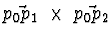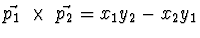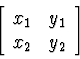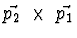Next: Up: Previous:

# Cross ProductAssuming p0 = (0, 0), compute the signed area within (0,0), p1, p2, p1 + p2 = (x1 + x2, y1 + y2)., the area of the parallelogram

This is the determinant of the matrixNote if p1 = (4,0) and p2 = (2,4), we are computing the signed area within (origin, p1, p2, p1 + p2) which is= 16 - 0 = 16.

If we computethe signed area is 0 - 16 = -16.Next: Up: Previous: